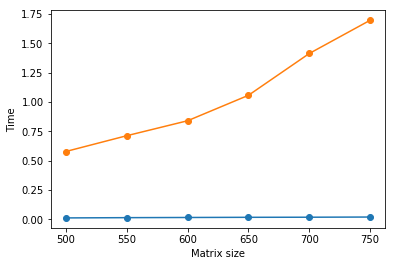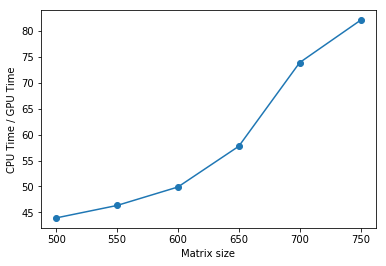# GPU is 40-80x faster than CPU in tensorflow for deep learning

1 min read

The speed difference of CPU and GPU can be significant in deep learning. But how much? Let’s do a test.

The computer:

The computer I use is a Amazon AWS instance g2.2xlarge (https://aws.amazon.com/ec2/instance-types/). The cost is \$0.65/hour, or \$15.6/day, or \$468/mo. It has one GPU (High-performance NVIDIA GPUs, each with 1,536 CUDA cores and 4GB of video memory), and 8 vCPU (High Frequency Intel Xeon E5-2670 (Sandy Bridge) Processors). Memory is 15G.

The script:

I borrowed Erik Hallstrom’s code from https://medium.com/@erikhallstrm/hello-world-tensorflow-649b15aed18c

The code runs matrix multiplication and calculate the time when using CPU vs GPU.

```from __future__ import print_function
import matplotlib
import matplotlib.pyplot as plt
import tensorflow as tf
import time

def get_times(maximum_time):

device_times = {
"/gpu:0":[],
"/cpu:0":[]
}
matrix_sizes = range(500,50000,50)

for size in matrix_sizes:
for device_name in device_times.keys():

print("####### Calculating on the " + device_name + " #######")

shape = (size,size)
data_type = tf.float16
with tf.device(device_name):
r1 = tf.random_uniform(shape=shape, minval=0, maxval=1, dtype=data_type)
r2 = tf.random_uniform(shape=shape, minval=0, maxval=1, dtype=data_type)
dot_operation = tf.matmul(r2, r1)

with tf.Session(config=tf.ConfigProto(log_device_placement=True)) as session:
start_time = time.time()
result = session.run(dot_operation)
time_taken = time.time() - start_time
print(result)
device_times[device_name].append(time_taken)

print(device_times)

if time_taken > maximum_time:
return device_times, matrix_sizes

device_times, matrix_sizes = get_times(1.5)
gpu_times = device_times["/gpu:0"]
cpu_times = device_times["/cpu:0"]

plt.plot(matrix_sizes[:len(gpu_times)], gpu_times, 'o-')
plt.plot(matrix_sizes[:len(cpu_times)], cpu_times, 'o-')
plt.ylabel('Time')
plt.xlabel('Matrix size')
plt.show()
plt.plot(matrix_sizes[:len(cpu_times)], [a/b for a,b in zip(cpu_times,gpu_times)], 'o-')
plt.ylabel('CPU Time / GPU Time')
plt.xlabel('Matrix size')
plt.show()```

Result:
Similar to Erik’s original finding, we found huge difference between CPU and GPU. In this test, GPU is 40 – 80 times faster than CPU.gpu_vs_cpu timecpu time / gpu time

Receive email notification via email 博客有新内容通知我
 Don't want to miss new papers in your field? Check out Stork we developed:专注医学生物类的论文润色联系他们请用优惠码 STORK4，他们会给你折扣。## Is AI able to write papers?

As researchers, we all have a dream: it would be fantastic if AI could write papers one day! This sounds like a science fiction,...

3 min read

1 min read

## Deep learning training speed with 1080 Ti and M1200

I compared the speed of Nvidia’s 1080 Ti on a desktop (Intel i5-3470 CPU, 3.2G Hz, 32G memory) and NVIDIA Quadro M1200 w/4GB GDDR5,...

1 min read